Characteristics and Structure of Matter
Perry Sprawls, Ph.D.Online Textbook Table of Contents
 INTRODUCTION AND OVERVIEW NUCLEAR STRUCTURE Composition Nuclides Isotopes Isobars Isomers Isotones NUCLEAR STABILITY Radioactivity NUCLEAR ENERGY ELECTRONS Energy Levels Concentration CHARACTERISTICS OF MATERIALS (Table)

# INTRODUCTION AND OVERVIEW

CONTENTS

Radiation is created and then later absorbed within some material substance or matter. Certain materials are more suitable than others as both radiation sources and absorbers. In this chapter we consider some basic physical characteristics of matter that determine how the materials interact with radiation. Radiation interactions, both formation and absorption, occur within individual atoms. We therefore begin with a brief review of atomic structure with an emphasis on atomic characteristics that affect interactions. We then discuss the collective properties of atoms within a material.

Atoms consist of two major regions: the nucleus and the electron shells. Each region has a role in radiation interactions. The nucleus is the source of energy for the radiations used in nuclear medicine procedures. Although the nucleus is not the source of x-ray energy, it is involved in the production of x-ray photons. In most instances radiation is absorbed by interacting with the electrons located in the shells surrounding the nucleus. Transitions in the shell electrons also produce one form of x-radiation.

# NUCLEAR STRUCTURE

CONTENTS

The conventional model of an atom consists of a nucleus containing neutrons and protons surrounded by electrons located in specific orbits or shells, as shown in below. The nucleus is shown as a ball or cluster of particles at the center of the atom. The nucleus is quite small in comparison to the total dimensions of the atom. However, most of the mass of the atom is contained within the nucleus. The components of the atom in the figure below are not drawn to scale. Actually, the electrons in the K, L, and M shells are much smaller than the protons and neutrons that make up the nucleus, and the electrons are located at a much greater distance from the nucleus than shown.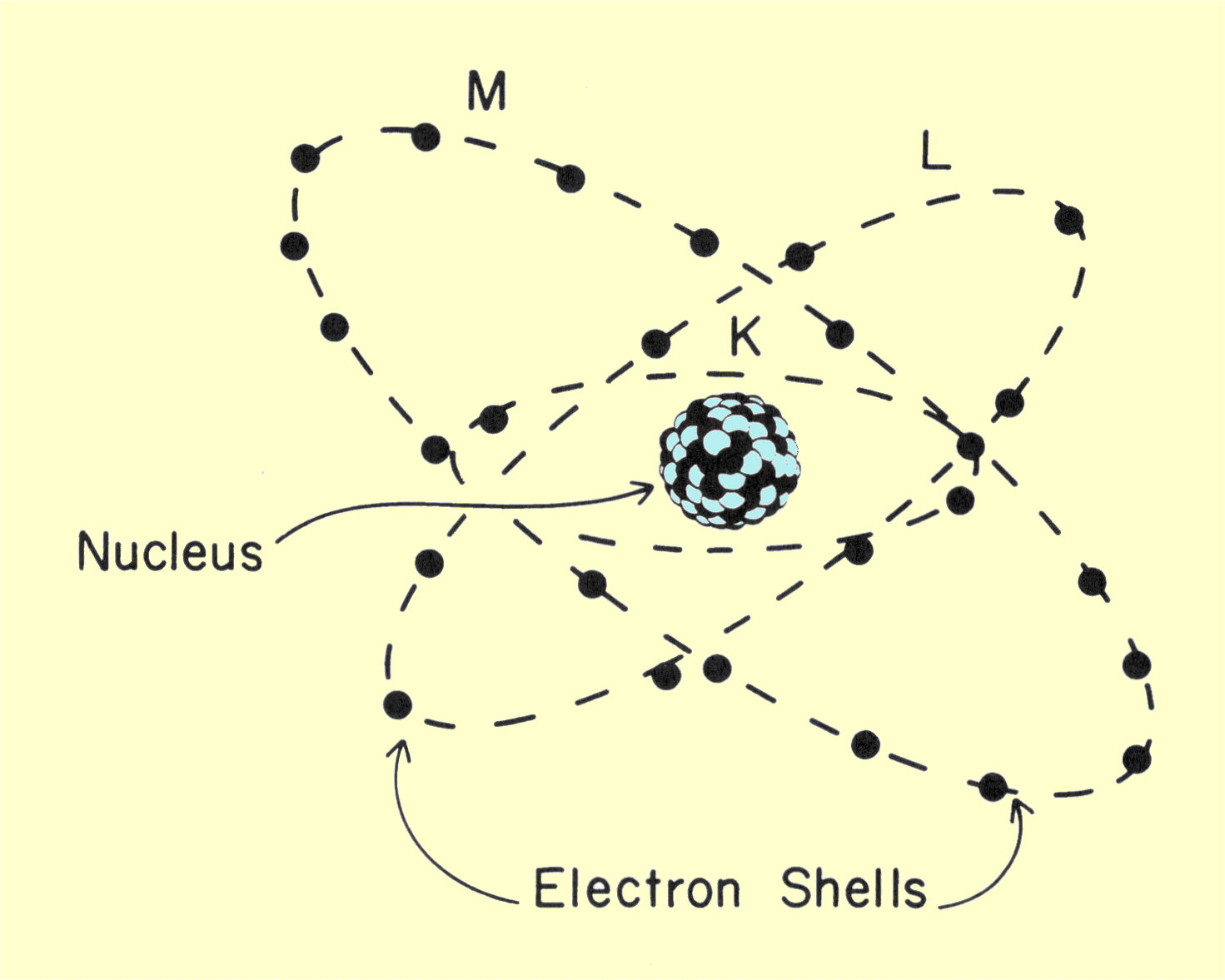# Composition

CONTENTS

All nuclei are composed of two basic particles, neutrons and protons. Neutrons and protons are almost the same size but differ in their electrical charge. Neutrons have no electrical charge and contribute only mass to the nucleus. Each proton has a positive charge equal in strength to the negative charge carried by an electron.

Most physical and chemical characteristics of a substance relate to the nuclei's neutron-proton composition. The number of protons in a nucleus is the atomic number (Z) and establishes the chemical identity of the atom. Each atomic number corresponds to a different chemical element; there are now approximately 106 known chemical elements that correspond to nuclei containing from 1 to 106 protons.

Because of their very small size it is not convenient to express the mass of nuclei and atomic particles in the conventional unit of kilograms. A more appropriate unit is the atomic mass unit (amu), the reference for which is a carbon atom with a mass number of 12, which is assigned a mass of 12.000 amu. The relationship between the atomic mass unit and kilogram is

1 amu = 1.66 x 10-27 kg.

The difference in mass between a neutron and proton is quite small: approximately 0.1 %. The larger difference is between the mass of these two particles and the mass of an electron. More than 1,800 electrons are required to equal the mass of a proton or neutron.

The total number of particles (neutrons and protons) in a nucleus is the mass number (A). Since neutrons and protons have approximately the same mass, the total mass or weight of a nucleus is, within certain limits, proportional to the mass number. However, the nuclear mass is not precisely proportional to the mass number because neutrons and protons do not have the same mass, and some of the mass is converted into energy when the nucleus is formed. The relationship between mass and energy is considered in more detail later.

There is a standard method for labeling different nuclear compositions: The mass number is designated by either a superscript preceding the chemical symbol, such as 14C or 131I, or by a number following the symbol, such as C-14, I-131, etc. The atomic number is added as a subscript preceding the chemical symbol. Adding the atomic number to the symbol is somewhat redundant since only one atomic number is associated with each chemical symbol or element.

With the exception of ordinary hydrogen, all nuclei contain neutrons and protons. The lighter elements (with low atomic and mass numbers) contain almost equal numbers of neutrons and protons. As the size of the nucleus is increased, the ratio of neutrons to protons increases to a maximum of about 1.3 neutrons per proton for materials with very high atomic numbers. The number of neutrons in a specific nucleus can be obtained by subtracting the atomic number from the mass number. One chemical element may have nuclei containing different numbers of neutrons. This variation in neutron composition usually determines if a nucleus is radioactive.

# Nuclides

CONTENTS

As stated previously, there are 106 different atomic numbers or elements. Since one element can have several different neutron numbers, there are obviously more than 106 different nuclear compositions. Actually, at least 1,300 different neutron-proton combinations are now known. The term element refers to the classification of a substance according to its atomic number, and the term nuclide refers to its classification by both atomic number and number of neutrons. In other words, whereas there are at least 106 different elements, there are about 1,300 different nuclides known.

The structural relationship of various nuclides is often shown on a grid generally referred to as a nuclide chart, as shown below. The scale in one direction represents the number of protons (atomic number), and the scale in the other direction represents the number of neutrons. Each square in the grid represents a specific nuclear composition or nuclide. Not all areas in the chart are occupied. Many neutron-proton combinations represent unstable combinations and do not exist as nuclei. The nuclides that do exist are located in a relatively narrow band that runs diagonally up through the chart.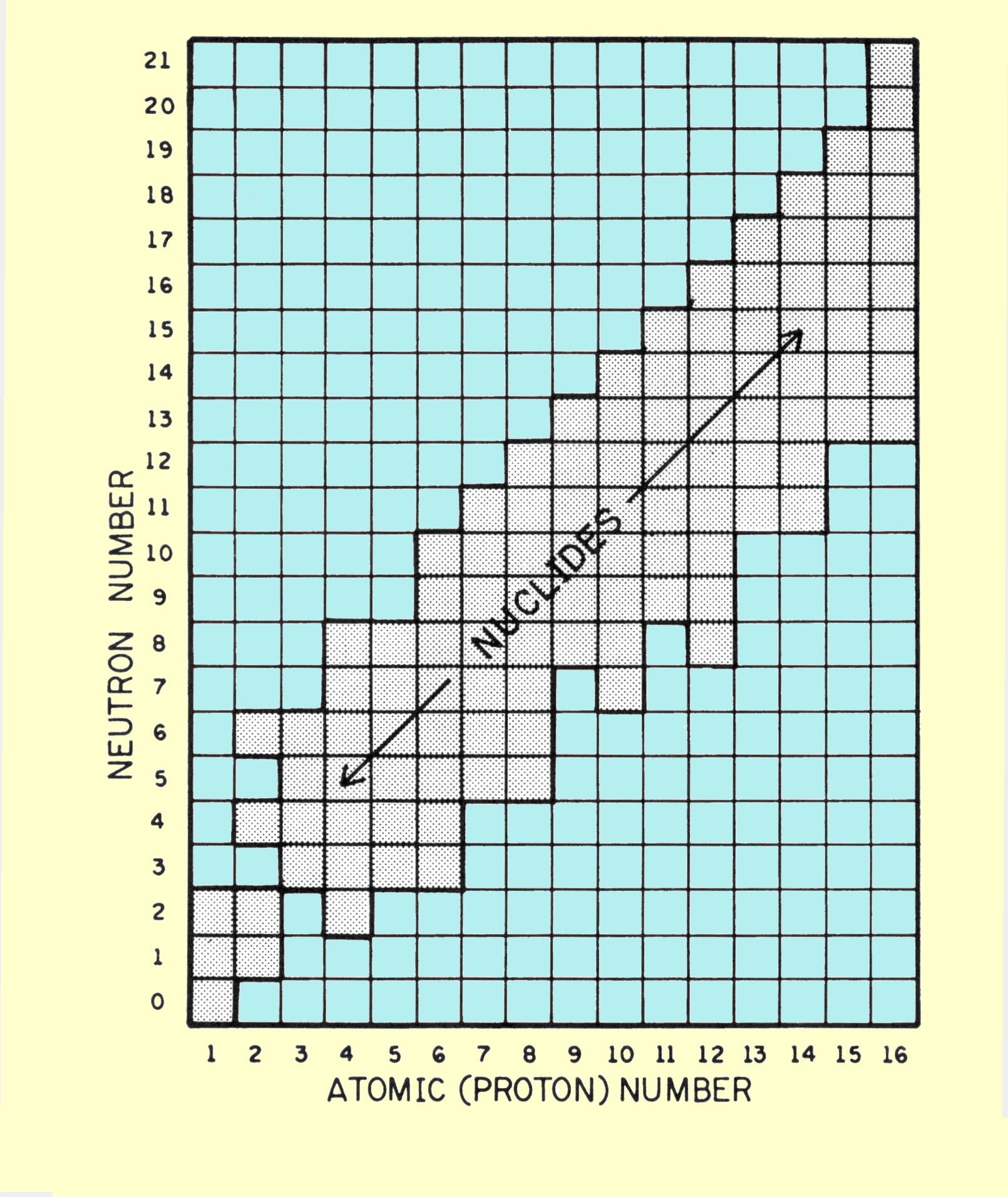Chart of Nuclides Arranged According to the Neutron-Proton Composition of the Nucleus

The charts in this text show only the first 16 elements because our immediate interest is in the characteristics of the chart, and not the details of all elements. Charts are published that show all known nuclides. Certain relationships between specific nuclides now need to be considered.

# Isotopes

CONTENTS

The nuclides of an element may contain different numbers of neutrons. Nuclides that belong to the same chemical element (and have the same atomic number) but have different numbers of neutrons are known as isotopes. It should be emphasized that the term isotope describes a relationship between or among nuclides rather than a specific characteristic of a given nuclide. An analogy is that persons who have the same grandparents but not the same parents are known as cousins. The isotopes of each element are located in the same vertical column of the nuclide chart as shown below.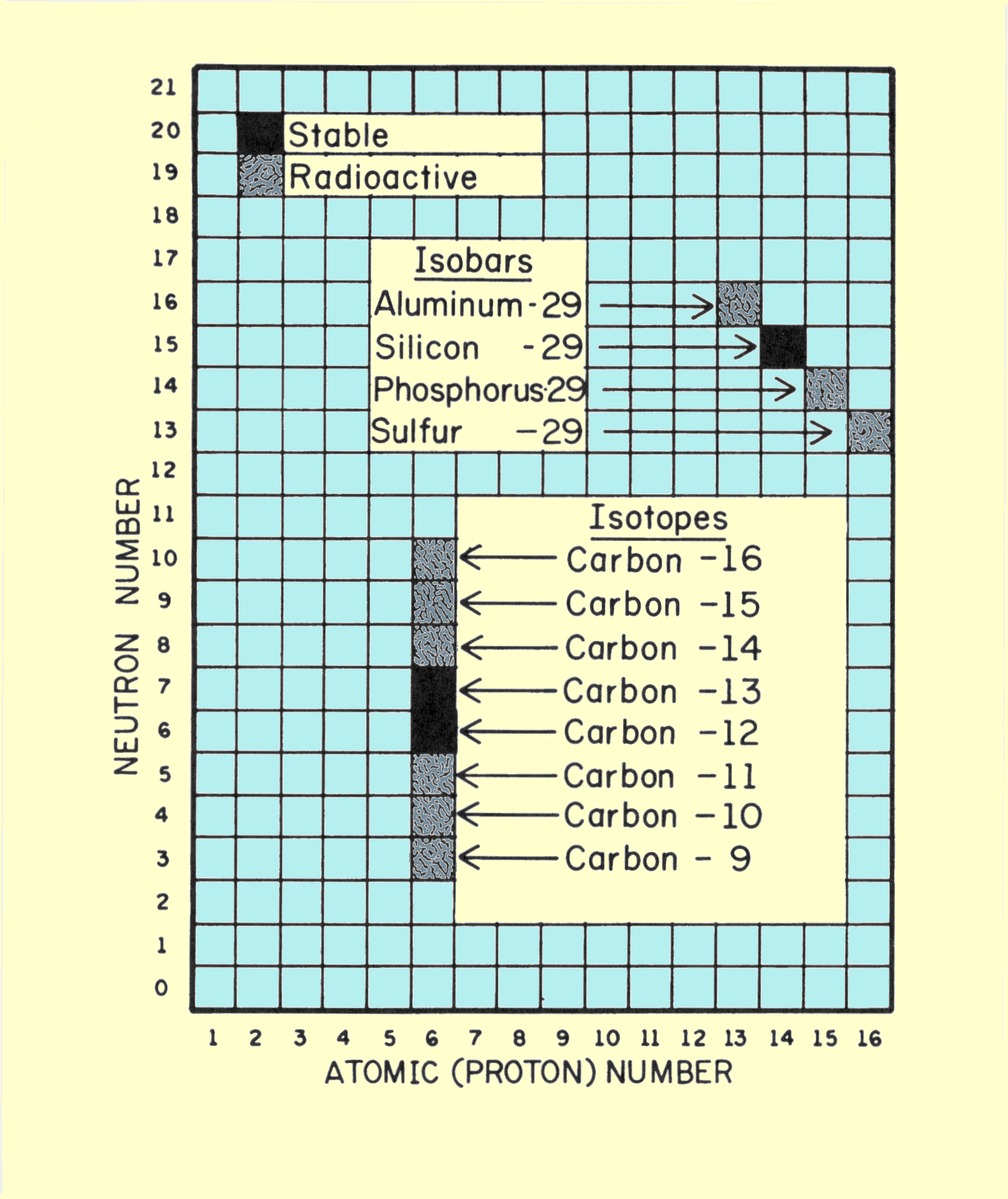Relationships among Isobars and Isotopes on a Nuclide Chart

There seems to be a general misconception that the term isotope means radioactive. This is obviously incorrect, since every nuclide is an isotope of some other nuclide. Most elements have several isotopes. In most cases some of the isotopes of a given element are stable (not radioactive), and some are radioactive. For example, iodine has 23 known isotopes with mass numbers ranging from 117 to 139. Two of these, I-127 and I-131, are shown below. The relationship between the two nuclides is that they are isotopes. I-131 is an isotope of I-127, and I-127 is also an isotope of I-131. For most elements the most common or most abundant form is the stable isotope. The radioactive forms are therefore isotopes of the more common forms, explaining the strong association isotopes have developed with radioactivity.

#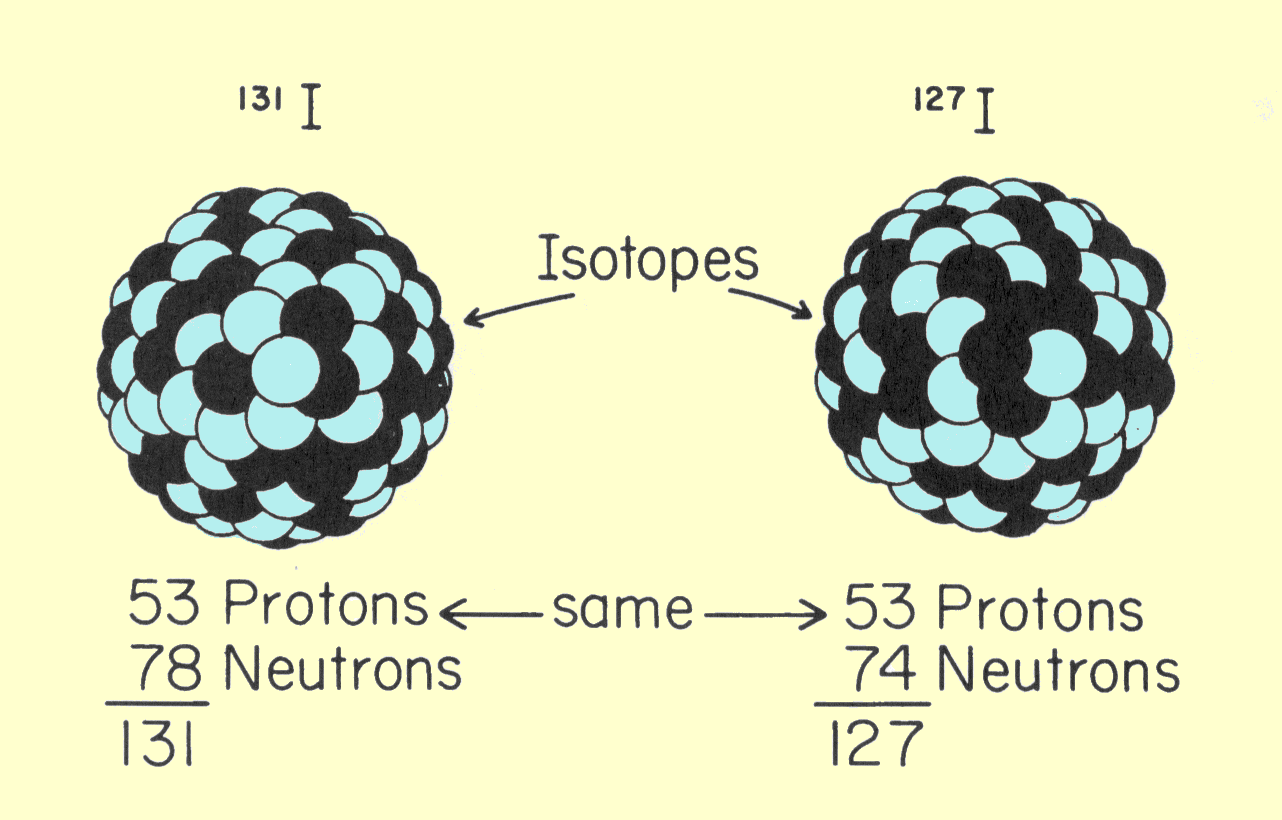Comparison of Two Isotopes

# Isobars

CONTENTS

Nuclides having the same mass number (total number of neutrons and protons) but different atomic numbers are known as isobars, as shown below.1-131 and Xe-131 are isobars of each other. A pair of isobars cannot belong to the same chemical element. The relationship among isobars in the nuclide chart (under "Isotopes" above), showing aluminum-29, silicon-29, phosphorus-29, and sulfur-29.

Our major interest in isobars is that in most radioactive transformations one nuclide will be transformed into an isobar of itself. For example, the I-131 shown in the figure immediately above is radioactive and is converted into Xe-131 when it undergoes its normal radioactive transformation.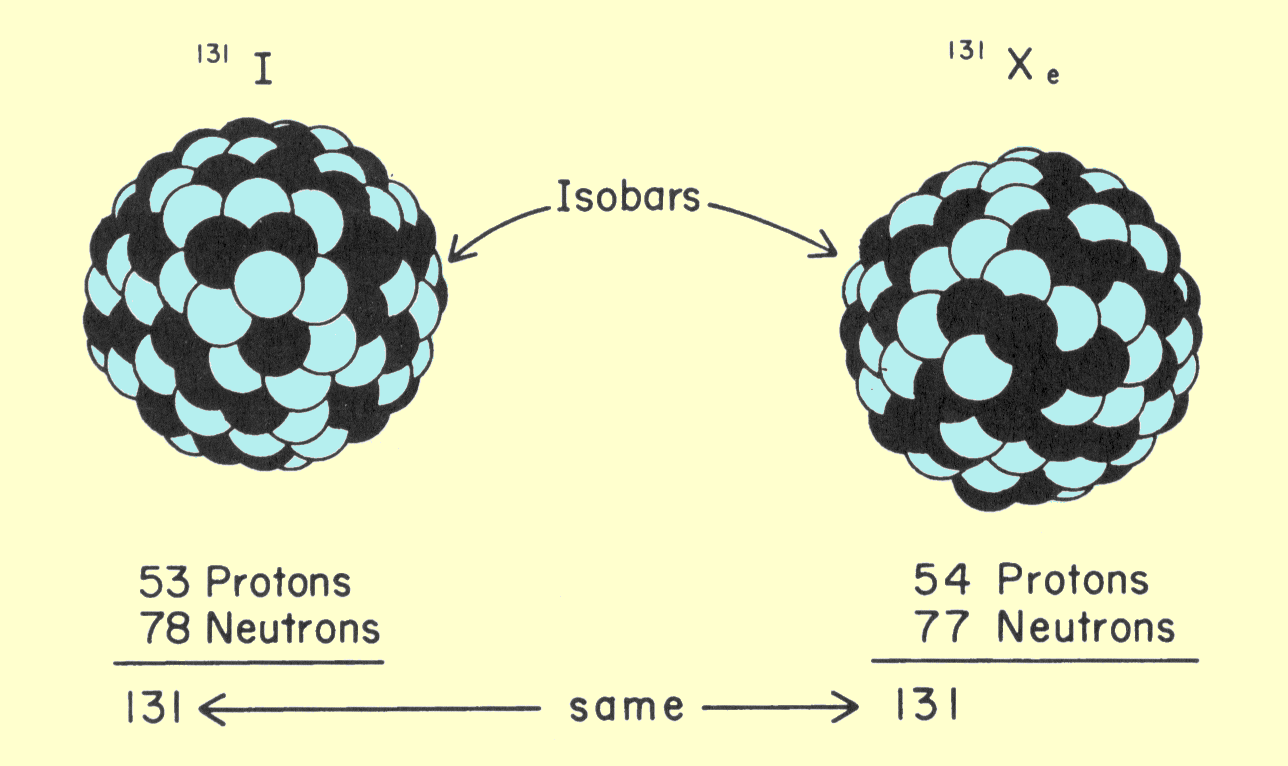Comparison of Two Isobars

# Isomers

CONTENTS

Nuclei can have the same neutron-proton composition but not be identical; one nucleus can contain more energy than the other. Two nuclei that have the same composition but varying energy are known as isomers. An example of a pair of isomers is shown below. Technetium-99 can exist in two energy states; the higher of the two is a temporary state generally referred to as a metastable state. The symbol for a nuclide in the metastable state is obtained by adding the letter m to the mass number (Tc-99m). A nucleus in the metastable state will eventually give off its excess energy and change to the other isomer. Such isomeric transitions have an important role in nuclear medicine and are discussed in detail later.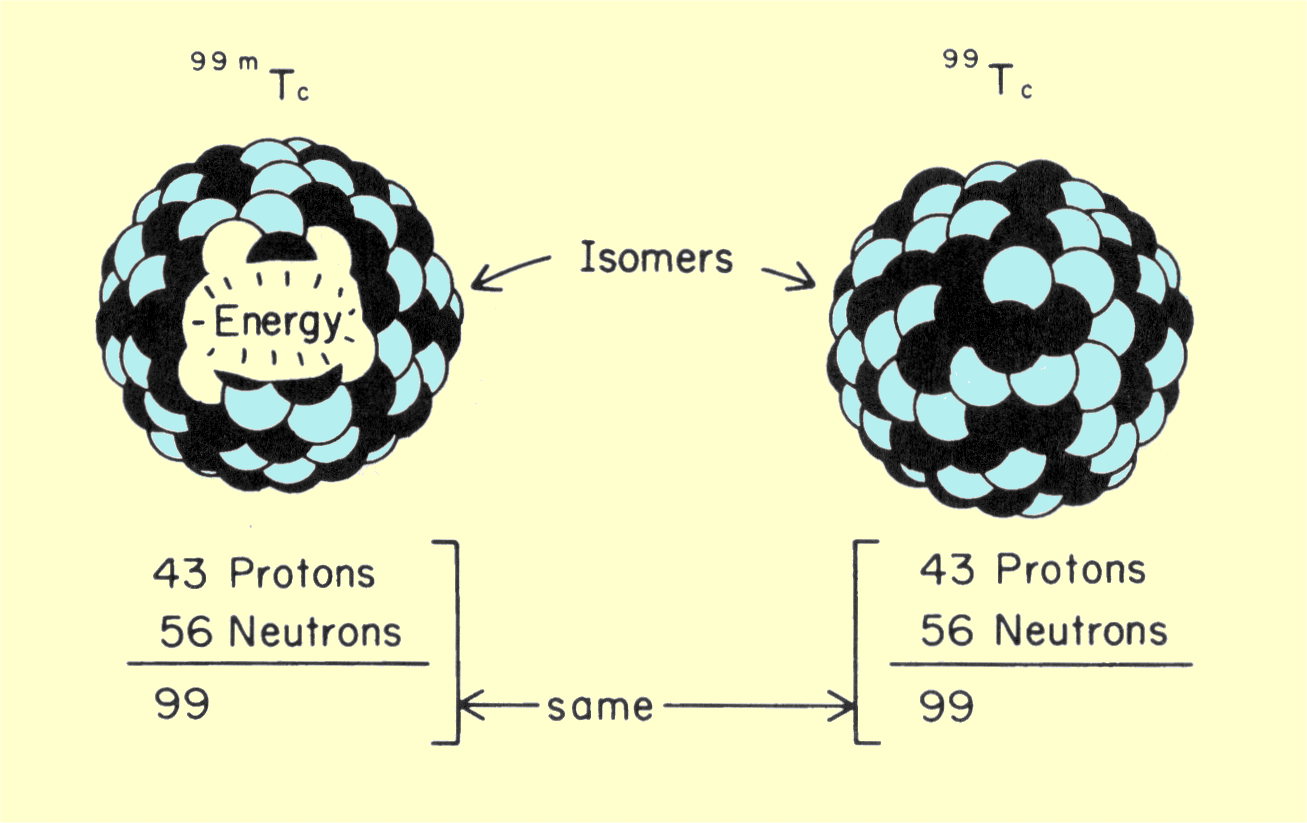# Isotones

CONTENTS

Nuclides that have the same number of neutrons are known as isotones. This relationship, mentioned here for the sake of completeness, is not normally encountered in nuclear medicine.

# NUCLEAR STABILITY

CONTENTS

The ability of a nucleus to emit radiation energy is related to its level of stability. The figure below illustrates three levels of nuclear stability. A stable nucleus will not undergo internal changes on its own. This is the condition of all non-radioactive material. The radioactive nuclei are stable enough to remain unchanged for a period of time, but will spontaneously undergo a transformation in which they will emit a burst of energy and become more stable; the second state is either a more stable radioactive state or a completely stable state. Many hypothetical nuclear compositions (neutron-proton mixtures) are completely unstable and cannot exist as intact nuclei. As stated previously, only about 1,300 different neutron-proton combinations, found among the stable and radioactive nuclides, will stick together as a nucleus.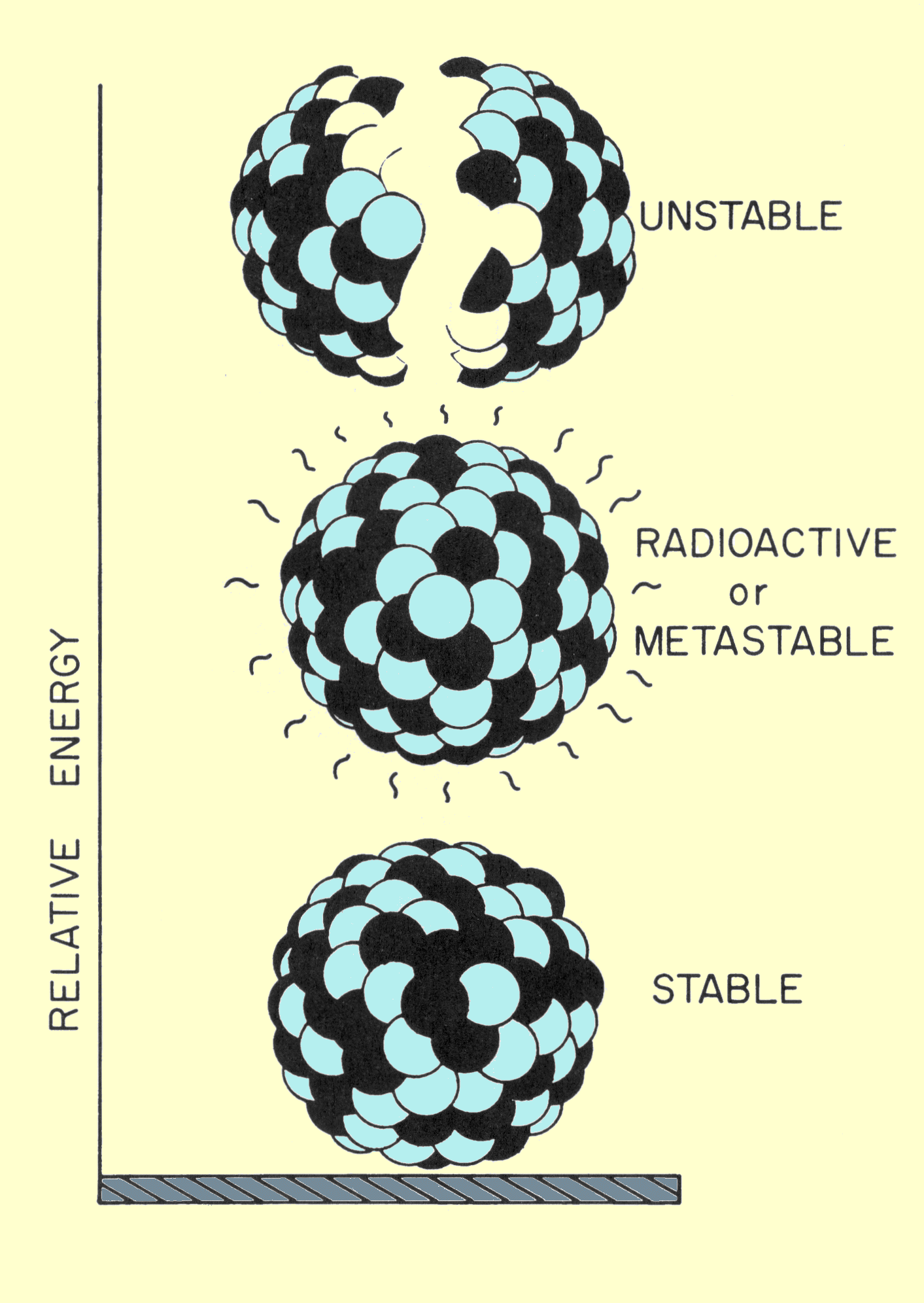The Three Levels of Nuclear Stability

Nuclear stability is determined by the balance of forces within the nucleus. There are forces that cause the nuclear particles (protons and neutrons) to be both attracted to and repelled from each other. Since each proton carries a positive electrical charge, protons repel each other. A short-range attraction force between all particles is also present within nuclei.

The most significant factor that determines the balance between the internal forces and therefore the nuclear stability is the ratio of the number of neutrons to the number of protons. For the smaller nuclei, a neutron-proton ratio of 1 to 1 produces maximum stability. The ratio for stability gradually increases with increasing atomic number up to a value of approximately 1.3 to 1.0 for the highest atomic numbers.

CONTENTS

If the neutron-proton ratio is slightly above or below the ratio for stability, the nucleus will generally be radioactive. Ratios considerably different from those required for stability are not found in nuclei because they represent completely unstable compositions. In an unstable composition, the repelling forces override the forces of attraction between the nuclear particles.

The relationship between nuclear stability and neutron-proton ratio is illustrated below. The stable nuclides, those with a neutron-proton ratio of approximately 1 to 1, are located in a narrow band running diagonally through the nuclide chart. The radioactive nuclides are located on either side of the stable band. All other areas on the nuclide chart represent neutron-proton mixtures that cannot exist as a nucleus.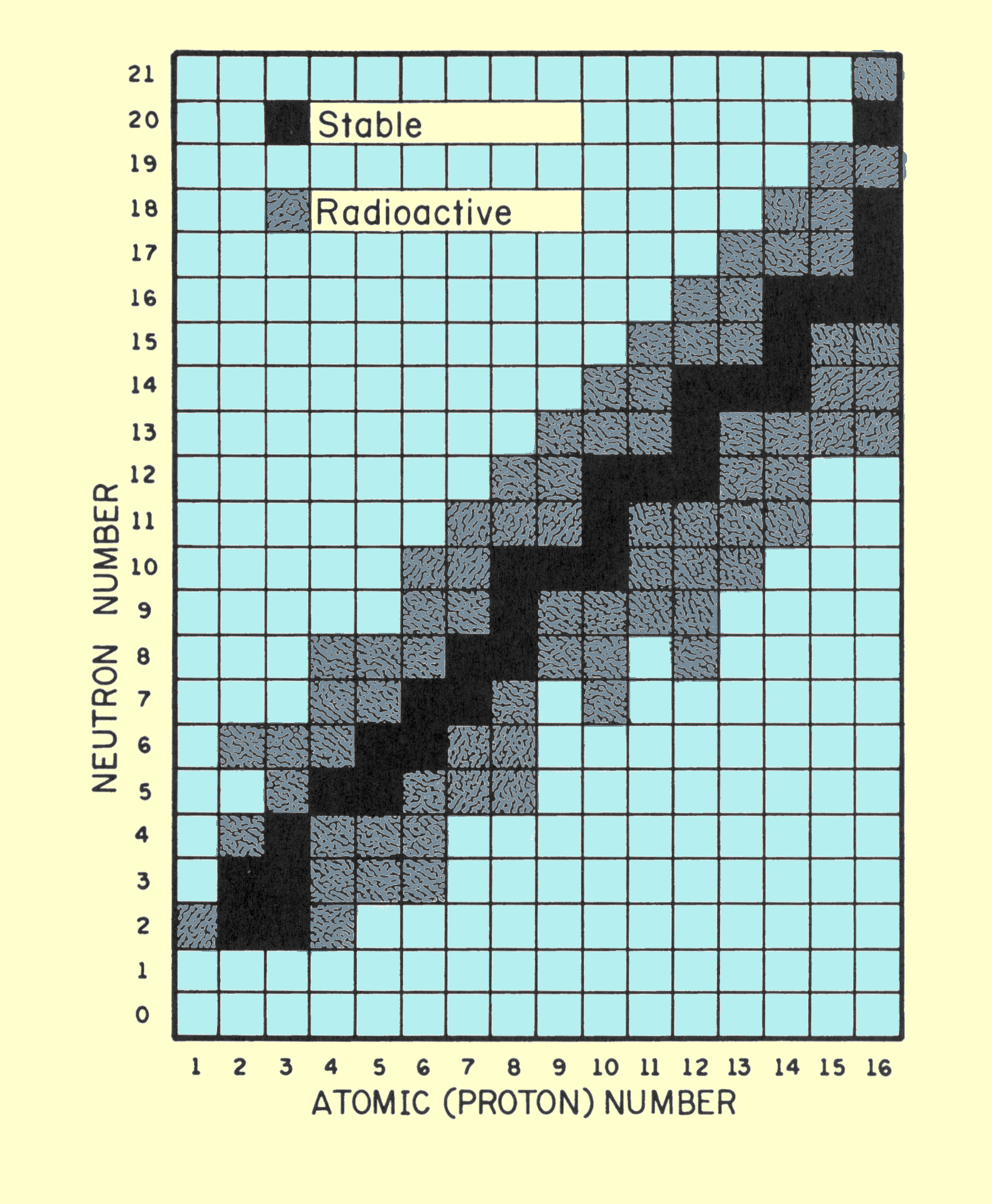Nuclide Chart Showing the Relationship of Unstable Radioactive and Stable Nuclear Structures

# NUCLEAR ENERGY

CONTENTS

Whenever a nucleus changes to a more stable form, it must emit energy. Several types of nuclear changes can result in the release of energy. Under certain conditions a nucleus can, by fission, break apart into more stable components. This process takes place in nuclear reactors where the energy released is often used to generate electrical energy. The fusion of two small nuclei to form a larger nucleus is the process that creates energy within the sun and the hydrogen bomb. In nuclear medicine, radiation energy is created when nuclei undergo spontaneous radioactive transitions to create more stable nuclear structures.

The energy emitted during nuclear transitions is created by converting a small fraction of the nuclear mass into energy. When such a conversion takes place, the relationship between the amount of energy (E) and the amount of mass (m) involved is given by Einstein's equation:

E = MC2

where c is the velocity of light. A significant aspect of this relationship is that a tremendous amount of energy can be created from a relatively small mass. The mass of 1 g, completely converted, would produce 25,000,000 kilowatt-hours.

In clinical applications we are interested in the amount of energy released by an individual atom. This is expressed in the unit of kiloelectron volts (keV), a relatively small unit of energy. The relationship between some other energy units and the keV are:

1 erg = 6.24 x 10
8 keV

1 j = 10
7 erg = 6.24 x 1015 keV.

The energy released in the radioactive transitions used in nuclear medicine is typically in the range of 100 keV to 500 keV. This corresponds to a nuclear mass change of 0.0001 amu to 0.0005 amu. The amount of nuclear mass used to produce the radiation energy is relatively small.

The energy equivalent of one electron mass is 511 keV. This value is often referred to as the rest-mass energy of an electron. In some situations in nuclear medicine procedures, the masses of individual electrons are completely converted into energy. The result is a photon with the characteristic energy of 511 keV.

# ELECTRONS

CONTENTS

Electrons are located in orbits or shells in the space surrounding a nucleus and have several important roles in image formation. In certain types of radioactive transitions, the orbital electrons become involved in the actual emission of energy from the atom. When radiation interacts with materials, such as human tissue, the interaction is usually with the electrons rather than the nuclei of the atoms.

# Atomic Number (Z)

CONTENTS

The number of electrons contained in a normal atom is equal to the number of protons in the nucleus. This number is the atomic number (Z) of the particular chemical element. Each electron has a negative electrical charge equivalent in strength to the positive charge of a proton. Under normal conditions, when the number of electrons and protons in an atom is the same, the positive and negative charges balance so that the atom has no net charge. However, if an electron is removed from an atom, the atom is said to be ionized and will have a positive charge.

# Energy Levels

CONTENTS

Electrons are located in discrete shells surrounding the nucleus, identified by letters of the alphabet beginning with K for the shell closest to the nucleus as shown above under "NUCLEAR STRUCTURE.". Each shell has a limited electron capacity. The maximum capacity of the K shell in any atom is 2 electrons; the L shell, 8 electrons; the M shell, 18 electrons; etc. The electron shells are generally filled beginning with the K shell and extending out until the total number of electrons have been placed.

Electrons are bound to the positive nucleus of an atom by their negative electrical charge. The strength of this binding can be expressed in terms of energy. This binding energy of an electron is equal to the amount of energy that would be required to remove the electron from the atom. Binding energy is a form of electron potential energy. As with any form of potential energy, some point must be designated the zero energy level. In the case of electrons, a location outside the atom where the electron is no longer under the influence of the nucleus is designated the zero point. Consider a golf ball resting on the ground. For the golf ball, the ground level would be the zero energy level. When an electron enters an atom, it drops to a lower energy level, just as the golf ball does when it rolls into a hole. Electrons within atoms are generally considered to have negative energy, since energy from some source must be added to raise them to the zero level. Likewise, the golf ball in the hole has negative energy with respect to the surface of the ground. It takes energy from some source to lift it back to zero level.

The concept of electron energy levels can be illustrated by using an energy level diagram of the type shown below. It should be noticed that this diagram represents the electrons as being down in a hole. The electrons near the bottom are the lowest energy level and would have the greatest binding energy.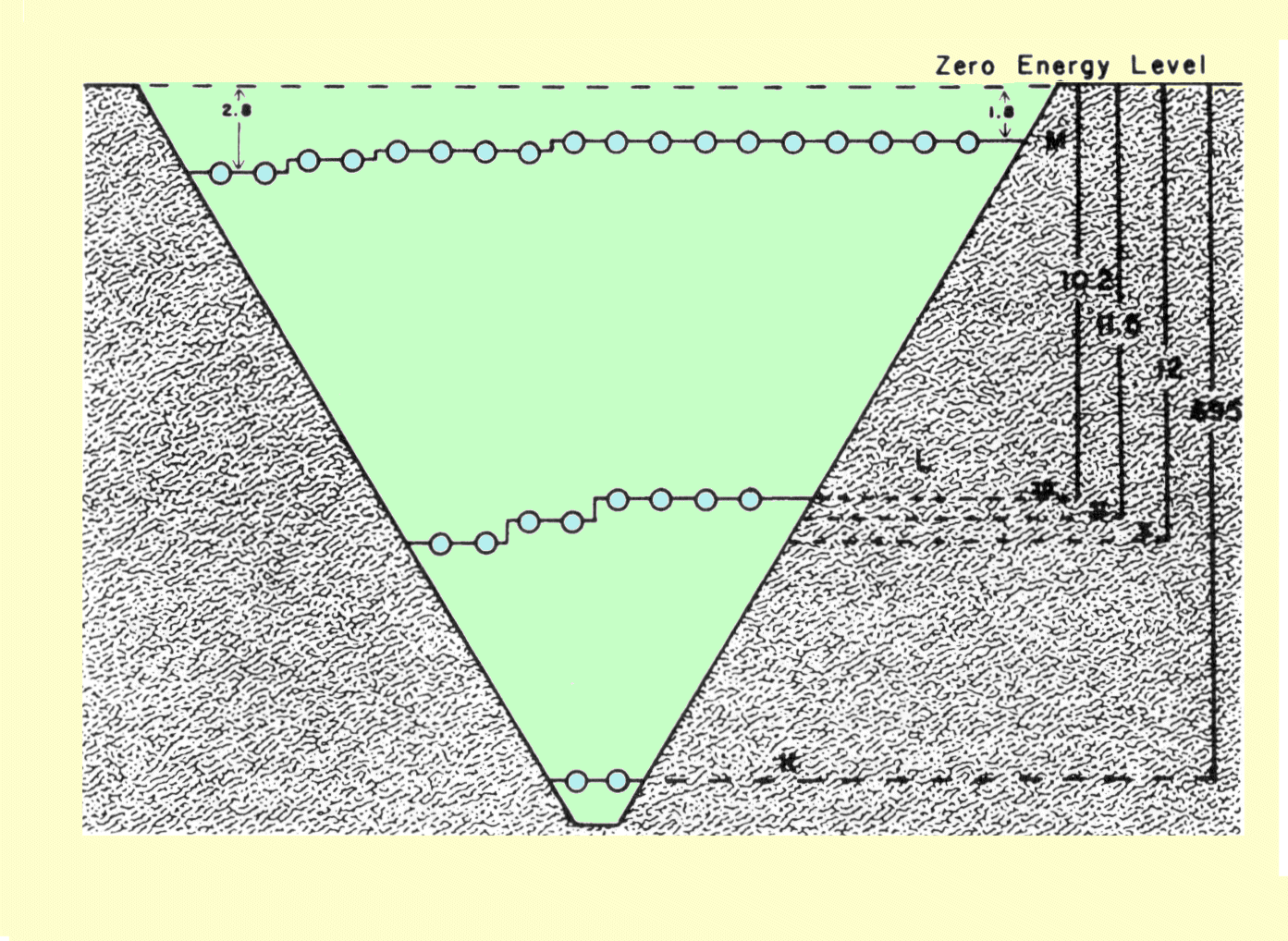Energy Level Diagram of Electrons within the Tungsten Atom

As discussed previously, the electrons are arranged within the atoms in definite layers, or shells. Each shell is a different energy level. The K shell, which is closest to the nucleus, is at the lowest energy level. The diagram above is for tungsten, which has an atomic number of 74. Only the K, L, and M electron levels are shown. Additional electrons are located in the N and O shells. These shells would be located above the M shell and slightly below the zero level. It should be noticed that there is a significant energy difference between the various shells. All of the shells, except K, are subdivided into additional energy levels. For example, the L shell is divided into three levels designated LI, LII, and LIII.

The roles of electrons in radiation events usually involve one of two basic principles: (1) Energy from some source is required to move an electron to a higher shell (such as K to L) or out of the atom; (2) If an electron moves to a lower shell (i.e., L to K), energy must be given up by the electron and usually appears as some type of radiation. The amount of energy involved depends on the difference in the energy levels between which the electrons move.

The binding energy for electrons in a specific shell, such as K, is related to atomic number as indicated in the figure below. It should be noticed that only the K-shell electrons for the higher atomic number elements have binding energies in the same range as the energies of diagnostic gamma and x-ray photons. This is significant in several types of interactions discussed later. The binding energy of the L-shell electrons is always much less than for the K, but it also increases with atomic number. For most substances, the binding energy of the outermost electrons is in the range of 5 eV to 20 eV. Obviously, these are the electrons that are the easiest to remove from an atom.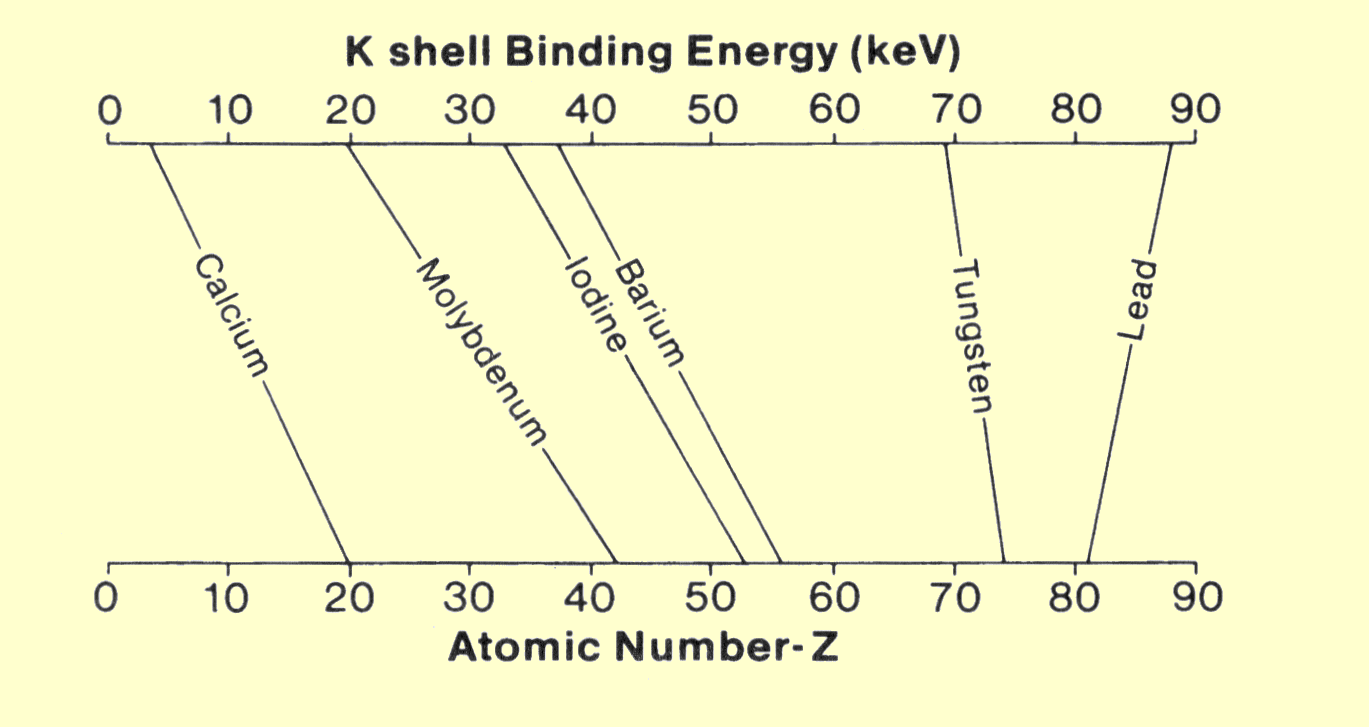Relationship between K-Shell Binding Energy and Atomic Number

The removal of an electron from an atom is called ionization. Since x-ray and gamma photons have sufficient energy to remove electrons from atoms, they can be considered ionizing radiation. Visible light, which has photon energies below the minimum binding energy in most atoms, cannot produce ionization.

# Concentration

CONTENTS

Photons are absorbed when they collide with electrons. As a photon passes through matter, its chances of being absorbed generally depend on the concentration of available electrons within the material. The concentration, or number of electrons per cubic centimeter, is given by

Electrons per cc =
rN(Z/A).

This relationship is the number of atoms per cubic centimeter multiplied by the atomic number, which is the number of electrons per atom. Several comments concerning this relationship are in order. Avogadro's number, N, always has the same value and obviously does not change from element to element. Z and A have unique values for each chemical element. It should be noticed, however, that the number of electrons per cubic centimeter depends only on the ratio of Z to A. The elements with lower atomic numbers have approximately one neutron for each proton in the nucleus. The value of Z/A is approximately 0.5. As the atomic number and atomic weight increase, the ratio of neutrons within the nucleus also increases. This produces a decrease in the Z/A ratio, but this change is relatively small. Lead, which has an atomic number of 82 and an atomic weight of 207, has a Z/A ratio of 0.4. For most material encountered in x-ray applications, the Z/A ratio varies by less than 20%. The single exception to this is hydrogen. Normal hydrogen contains no neutrons and has a nucleus that consists of a single proton. The Z/A ratio, therefore, has a value of l.

Since Avogadro's number is constant, and the Z/A ratio is essentially constant, the only factor that can significantly alter the electron concentration is the density of the material. Most materials, especially pure elements, have more or less unique density values. In compounds and mixtures, the density depends on the relative concentration of the various elements.

The fact that electron concentration does not significantly change with atomic number might suggest that atomic number has little to do with electron-x-ray interactions. This is, however, not the case. As x-ray photons pass through matter, the chance of interaction depends not only on electron concentration, but also on how firmly the electrons are bound within the atomic structure. Certain types of interactions occur only with firmly bound electrons. Since the binding energy of electrons increases with atomic number, the concentration of highly bound electrons increases significantly with increased atomic number.

Atomic number is essentially a characteristic of the atom and has a value that is unique to each chemical element. Many materials, such as human tissue, are not a single chemical element, but a conglomerate of compounds and mixtures. With respect to x-ray interactions, it is possible to define an effective atomic number, Z
eff, for compounds and mixtures. The effective atomic number is given by

___________________________
Zeff = 2.94f1Z12.94 + f2Z22.94 + f3Z32.94 + - - .

In this relationship, f is the fraction of the total number of electrons associated with each element. The exponent, 2.94, is derived from the relationship between x-ray interactions and atomic number, which is discussed later.

Water, which is a major component of the human body, can be used to demonstrate the concept of effective atomic number. The water molecule contains two hydrogen atoms, which have one electron each, and one oxygen atom with eight electrons. The electron fractions, f, are therefore 0.2 for hydrogen and 0.8 for oxygen. Substitution of these values in the above relationship gives an effective atomic number for water of

______________________
Z =
2.94(0.2 x 1)2.94 + (0.8 x 8)2.94

= 7.42.

In many x-ray systems, a variety of materials are involved in x-ray interactions. Many of these materials are listed in the following table, along with their principal physical characteristics and relationship to the x-ray system.

# CHARACTERISTICS OF MATERIALS

CONTENTS

 Material Atomic number* (Z) K electron binding energy (keV) Density (g/cc) Application Beryllium 4.0 - - 1.85 Low absorbing tube window Fat 5.92 - - 0.91 Body tissue Water 7.42 - - 1.0 Tissue "equivalent" Muscle 7.46 - - 1.0 Body tissue Air 7.64 - - 0.00129 - - Aluminum 13.0 - - 2.7 X-ray filter and pene- tration reference Bone (femur) 14.0 - - 1.87 Body tissue Calcium 20.0 - - 1.55 Body deposits Copper 29.0 8.9 8.94 X-ray filter Molybdenum 42.0.0 20.0 10.22 X-ray source Silver 47.0 25.5 10.5 Absorber in film Iodine 53.0 33.2 4.94 Contrast media and receptor absorber Xenon 54.0 34.5 0.0059 Receptor absorber Barium 56.0 37.4 3.5 Contrast media and receptor absorber Lanthanum 56.0 38.9 6.15 Receptor absorber Gadolinium 64.0 50.2 7.95 Receptor absorber Tungsten 74.0 69.5 19.3 X-ray source Lead 82.0 88.0 11.34 X-ray absorber for shielding

*Effective Z of tissues from Spiers (1946).
Source: Spiers FW: Effective atomic number and energy absorption in tissues. Br J Radiol  1946; 19:218.